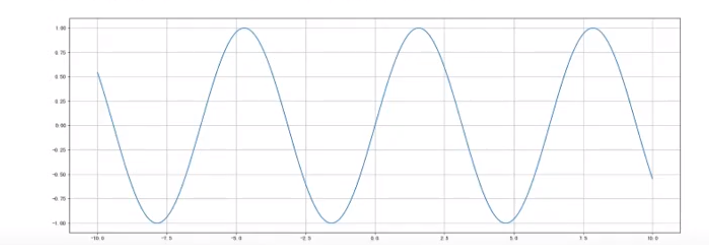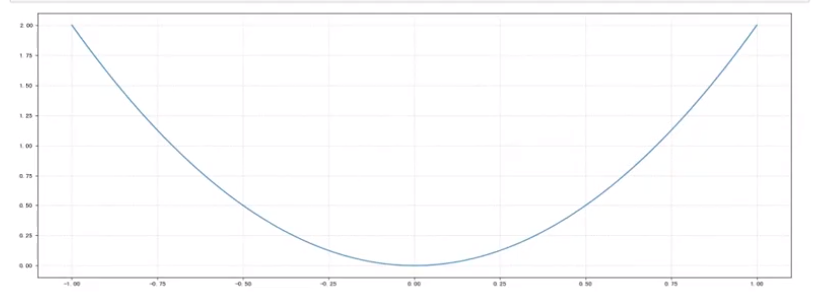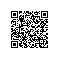# 折线图的应用场景

• 呈现公司产品（不同区域）每天活跃用户
• 呈现app每天下载数量
• 呈现产品新功能上线后，用户点击次数随时间的变化
• 拓展：画各种数学函数图像

• 注意：plt.plot()除了可以画折线图，也可以画各种数学函数图像。import numpy as np

#1)准备数据
x=np.Linspace(-10， 10， 1000)
y=np.sin(x)

#2)创建画布
plt， figure(fig size=(20， 8) ， dpi=100)

#3)绘制函数图像
plt.plot(x， y)

#添加网格显示
plt.grid()

import numpy as np

#1、准备x y数据
x=np.linspace (-1,1,1000)
y=2 * x * x

#2、创建画布
plt， figure(fig size=(20， 8) ， dpi=80)

#3、绘制函数图像
plt.plot(x， y)

#添加网格显示
plt.grid(linestyle="--",alpha=0.5)

#4、显示图像
plt.show()### 配套视频课程点击这里查看Python学习站使用钉钉扫一扫加入圈子
+ 订阅

Python学习资料大全，包含Python编程学习、实战案例分享、开发者必知词条等内容。